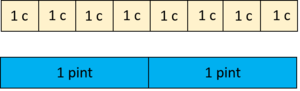# Many Ways to Say It

Alignments to Content Standards: 6.RP.A.1

## Paint

Abigail mixed 2 cups of white paint with 6 tablespoons of blue paint. Which of the following statements describes this situation?1. There is 1 cup of white paint for every 3 tablespoons of blue paint.
2. The ratio of the number of cups of white paint to the number of tablespoons of blue paint is 1:3.
3. There are 3 tablespoons of blue paint per cup of white paint.
4. For each tablespoon of blue paint there are 3 cups of white paint.

## Paste

Clara mixed 8 cups of flour with 2 pints of water to make papier-mâché paste. Describe the relationship between flour and water in the paste in at least four different ways.

## IM Commentary

The purpose of this task is to help students understand and use ratio language. The picture can help them make sense of any unfamiliar language. A teacher could ask, "Do any of these make sense? How can you make sense of it using the picture?" Students may need the teacher to make it explicit that "per" means "for every one."

Note that two of the possible wordings for the paste use fractions. Students are not expected to do this (yet) but if they do, it is correct. The teacher may wish to highlight them in anticipation of work to come later.

## Paint

The first three of the statements describe this situation:

1. There is 1 cup of white paint for every 3 tablespoons of blue paint.
2. The ratio of the number of cups of white paint to the number of tablespoons of blue paint is 1:3.
3. There are 3 tablespoons of blue paint per cup of white paint.

## PasteHere are 12 ways to describe the relationship between the flour and water using ratio or rate language. Any equivalent ratio is also acceptable.

1. There are 8 cups of flour for every 2 pints of water.
2. There are 2 pints of water for every 8 cups of flour.
3. There are 4 cups of flour for every 1 pint of water.
4. There is 1 pint of water for every 4 cups of flour.
5. The ratio of the number of cups of flour to the number pints of water is 8:2.
6. The ratio of the number of cups of flour to the number pints of water is 4:1.
7. The ratio of the number pints of water to the number of cups of flour is 2:8.
8. The ratio of the number pints of water to the number of cups of flour is 1:4.
9. There are 4 cups of flour per pint of water.
10. There is $\frac14$ pint of water for every 1 cup of flour.
11. For each pint of water there are 4 cups of flour.
12. For each cup of flour there is $\frac14$ pint of water.